# 不可压缩Navier-Stokes方程最优动力系统建模和分析*

(1. 大连理工大学 航空航天学院， 辽宁 大连 116024; 2. 北京应用物理与计算数学研究所， 北京 100088)

## 引 言

POD基有许多很好的性质.首先，从数学的角度，POD基被证明是均方意义(能量)下的最优降维方法，因此POD基能够客观地逼近未知流动数据库.其次，根据惯性流形能够掌控变量的思想，高阶模态的影响可以被低阶模态包含.并且，用POD方法来降维偏微分方程可以抑制数值不稳定现象和节省计算资源[9-12].然而，有必要指出POD方法的三个缺陷: 第一，此方法只能被用于内积型最优化问题；第二，投影误差无法被避免；第三，POD基必须从已知的数据库中获得.

## 1 最优动力系统建模理论基础

### 1.1 一般理论框架(1)

u(x,t)∈Wm,p(ΩT),

(2)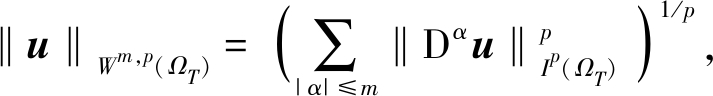(3)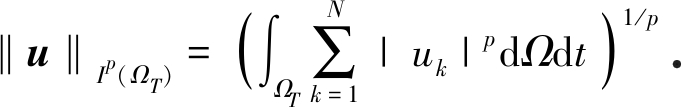(4)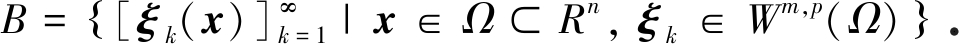(5)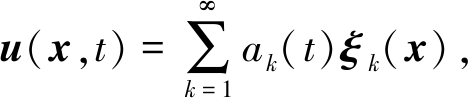(6)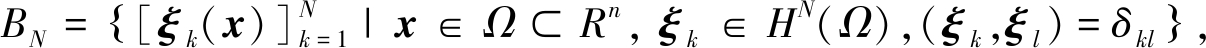(7)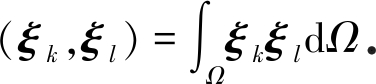(8)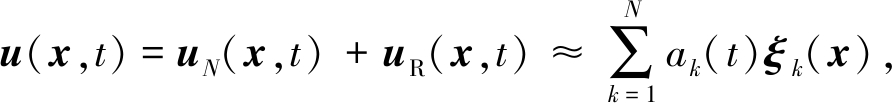(9)

ak=(u,ξk).

(10)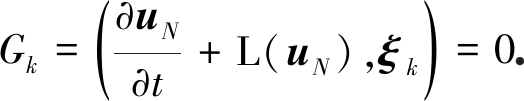(11)

Galerkin投影方程组是方程(1)的低维模型，也被称为低维动力系统，可以写为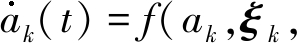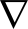ξk,…),

(12)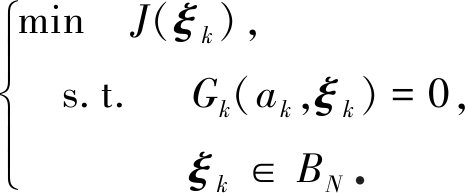(13)

1) 函数空间BBN的类型；

2) 目标泛函J(ξk)的具体形式；

3) 具体问题，包括控制方程、初始条件和边界条件；

4) 截断阶数N.

### 1.2 广义目标泛函及最优动力系统模型方程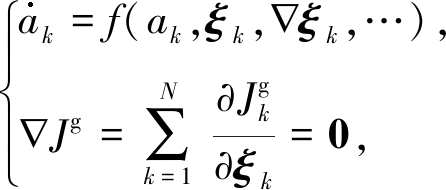(14)

## 2 不可压缩Navier-Stokes方程最优动力系统建模与分析

### 2.1 建模理论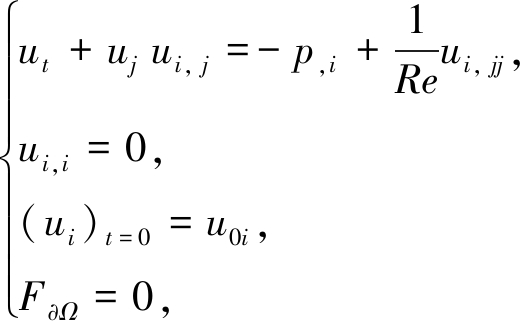(15)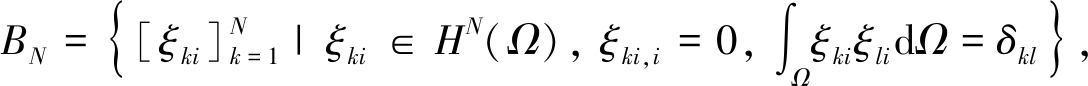(16)

ξki二阶可微.

ui在函数空间BN中的低维近似表达式为

ui=ak(t) ξki(xi)+uRiak(t) ξki(xi)，

(17)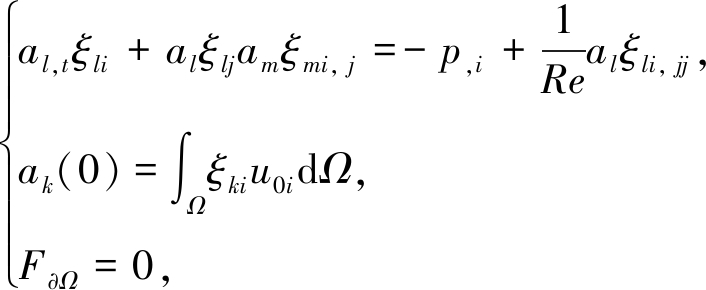(18)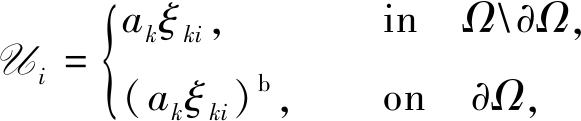(19)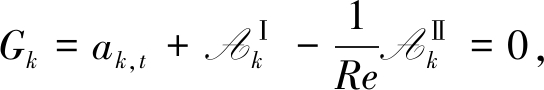(20)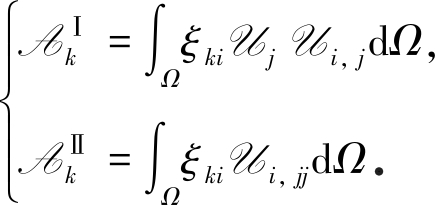(21)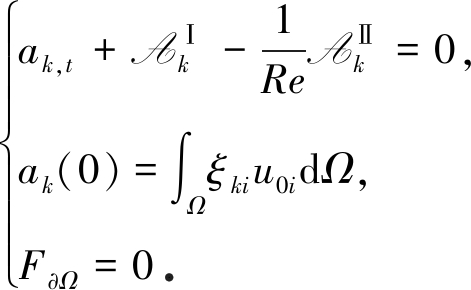(22)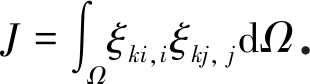(23)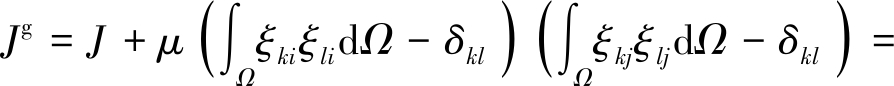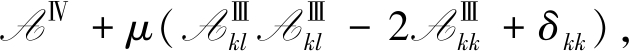(24)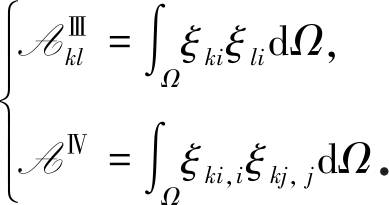(25)

1) 关于ak,t的动力系统方程(26)

2) 广义目标泛函梯度方程

Ω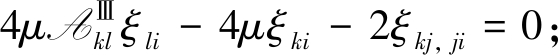(27)

ξkj, jni=0,

(28)

### 2.2 速度边界条件的数值实现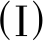滑移边界条件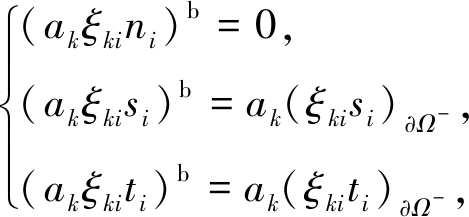(29)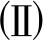无滑移边界条件

(akξki)b=0.

(30)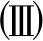入流边界条件

(akξki)b=ubi

(31)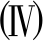连续出流边界条件

(akξki)b=ak (ξki)Ω-.

(32)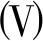周期边界条件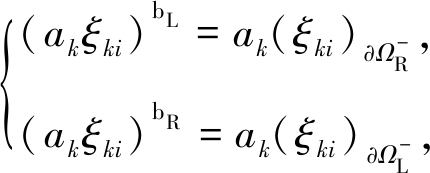(33)

1) 左边界 入流边界和无滑移边界的混合；

2) 右边界 连续出流边界；

3) 前后上下边界 滑移边界.

## 3 最优动力系统的数值分析

### 3.1 数值算例和POD分析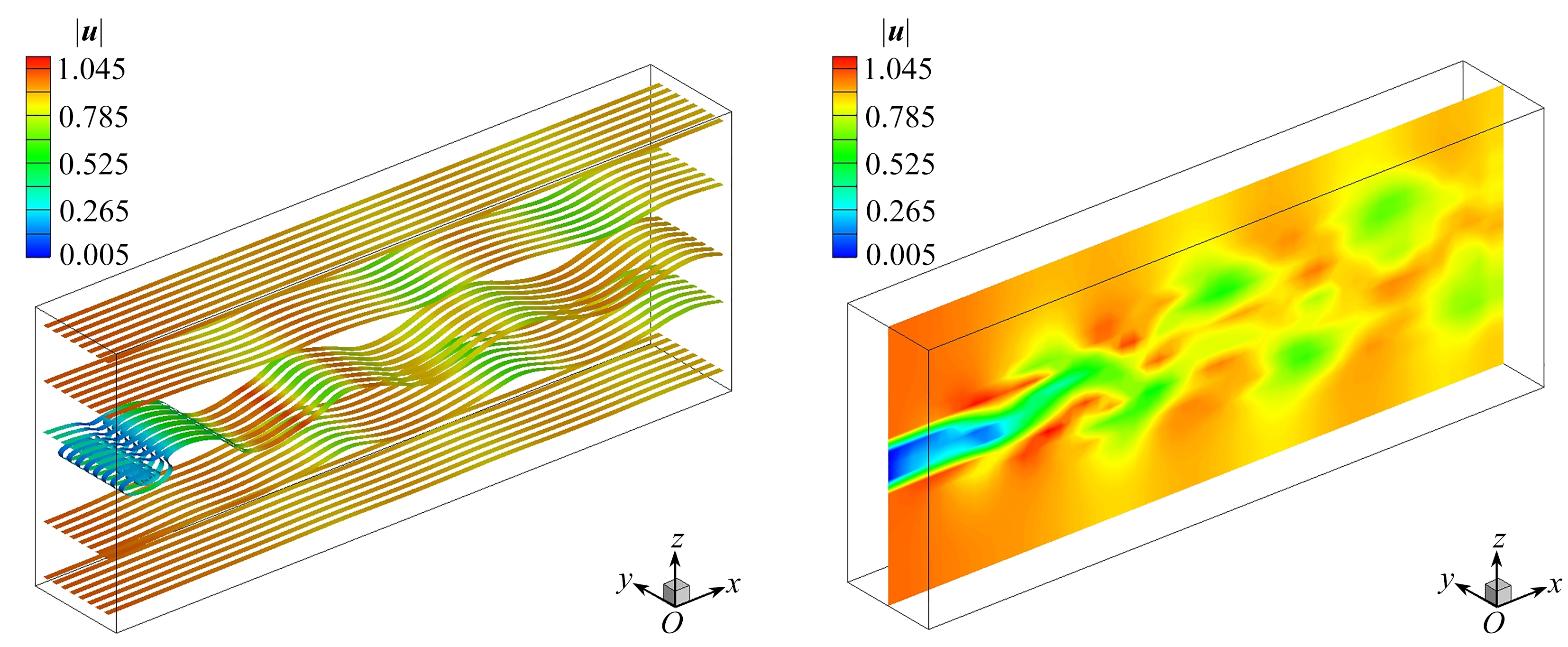(a) 带状流线 (b) 速度模值云图 (a) Ribboned streamlines(b) The velocity contour

Fig. 1 The coarse velocity field for t=700.0

Table 1 The energy distribution of POD bases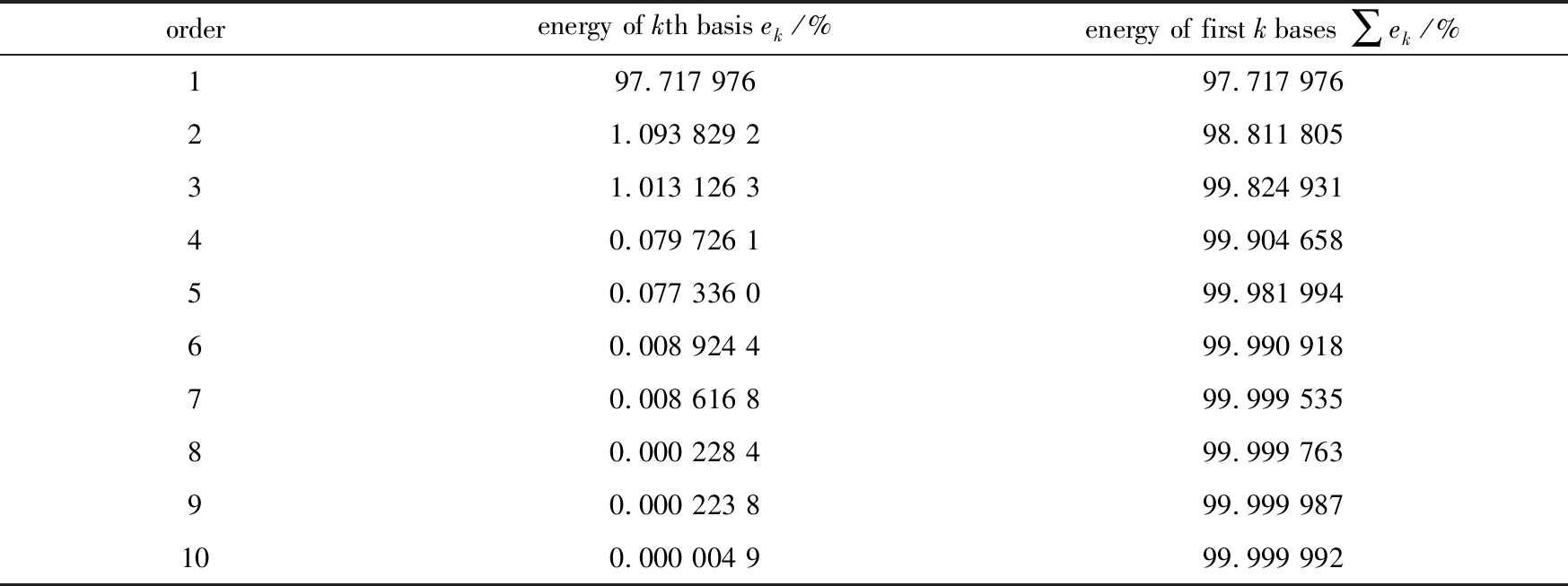orderenergy of kth basis ek/%energy of first k bases ∑ek/%197.717 97697.717 97621.093 829 298.811 80531.013 126 399.824 93140.079 726 199.904 65850.077 336 099.981 99460.008 924 499.990 91870.008 616 899.999 53580.000 228 499.999 76390.000 223 899.999 987100.000 004 999.999 992

### 3.2 全局最优化与最优基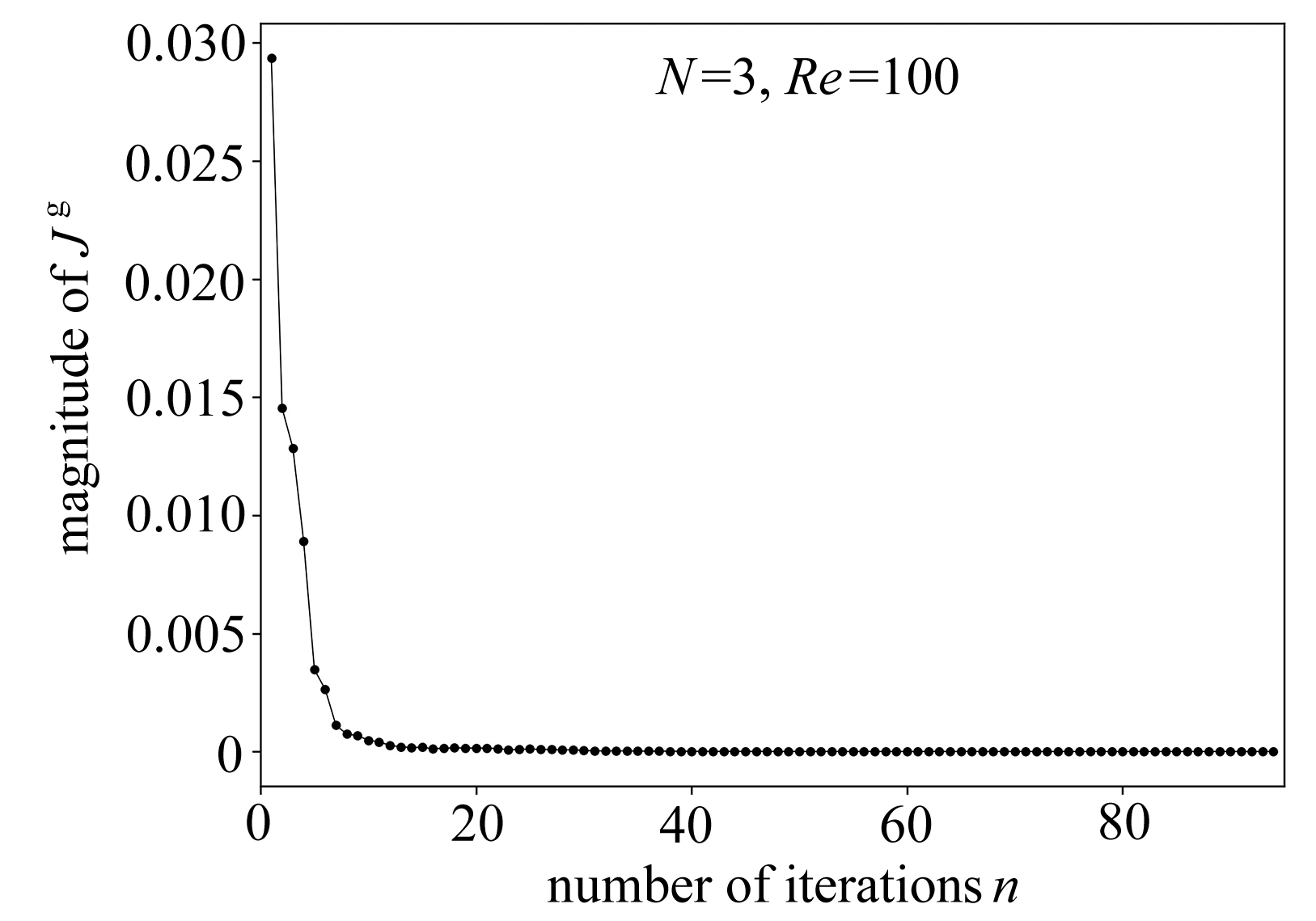Fig. 2 The convergence curve of Jg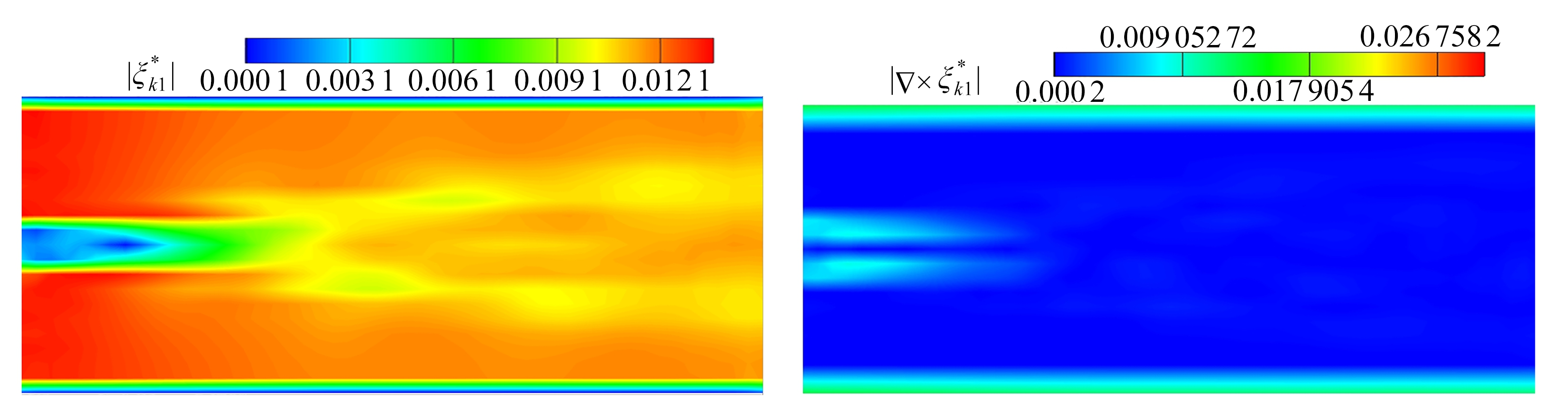(a) 第一阶最优基模值 (b) 第一阶最优基旋度模值 (a) The 1st-order optimal basis (b) The 1st-order optimal basis curls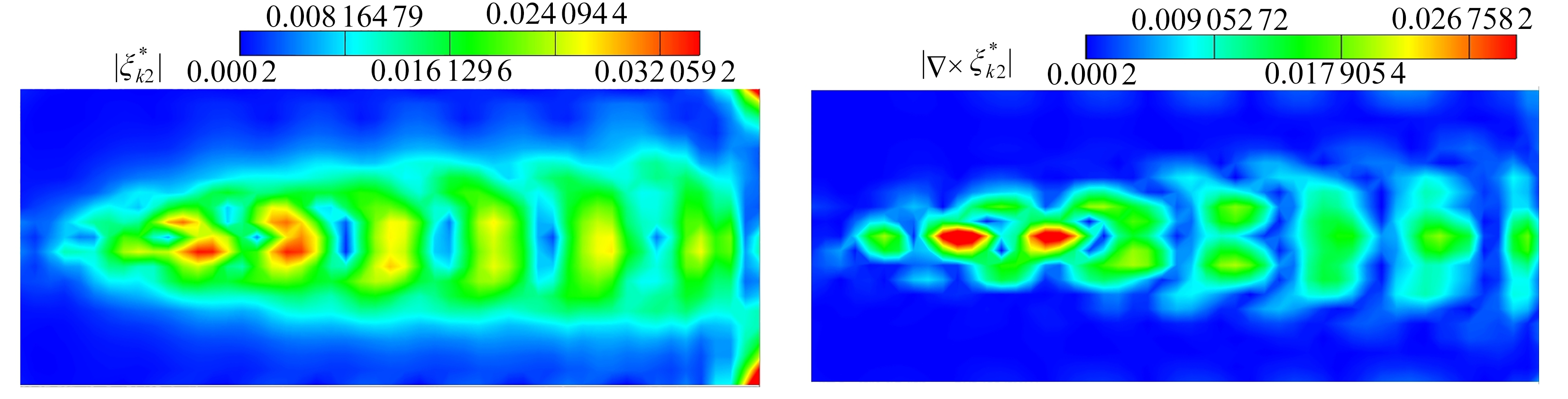(c) 第二阶最优基模值 (d) 第二阶最优基旋度模值 (c) The 2nd-order optimal basis (d) The 2nd-order optimal basis curls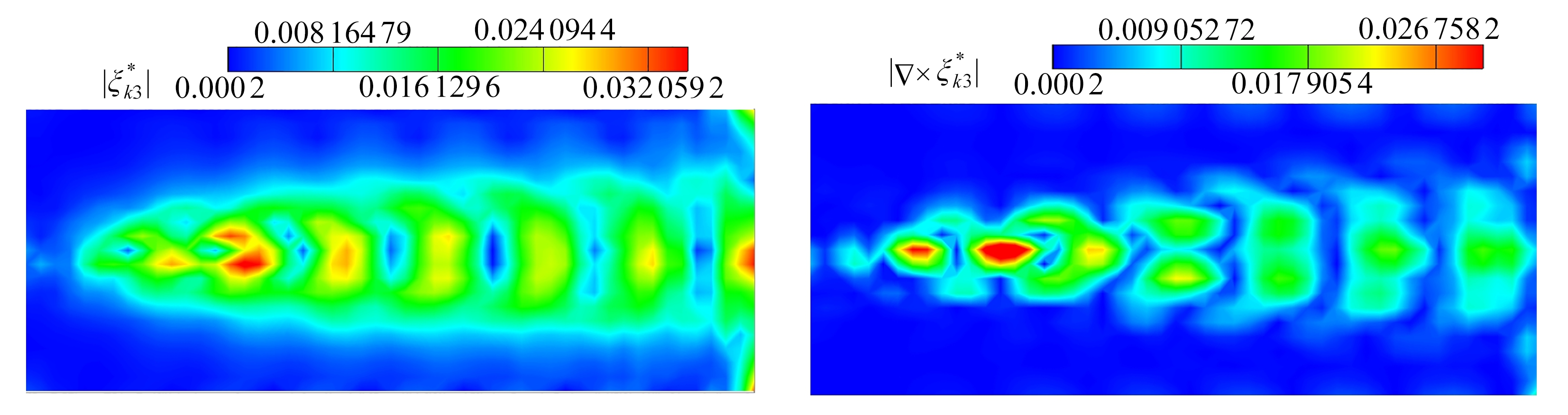(e) 第三阶最优基模值 (f) 第三阶最优基旋度模值 (e) The 3rd-order optimal basis (f) The 3rd-order optimal basis curls

Fig. 3 The contours of the optimal basis magnitudes and curls in the streamwise middle section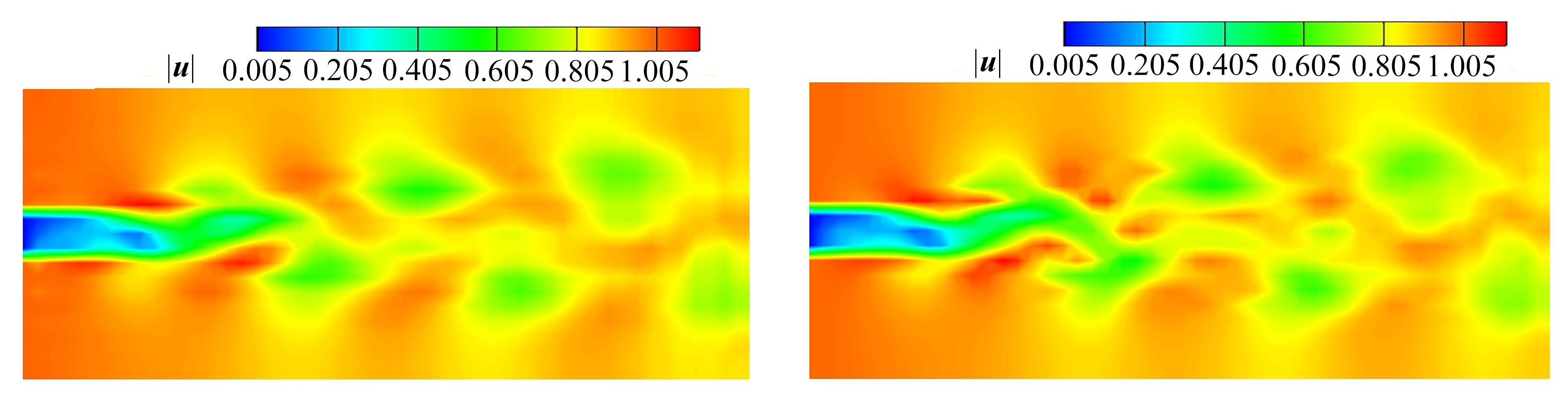(a) t=700.0最优动力系统流场 (b) t=700.0数据库流场 (a) The optimal dynamical system for t=700.0 (b) The database for t=700.0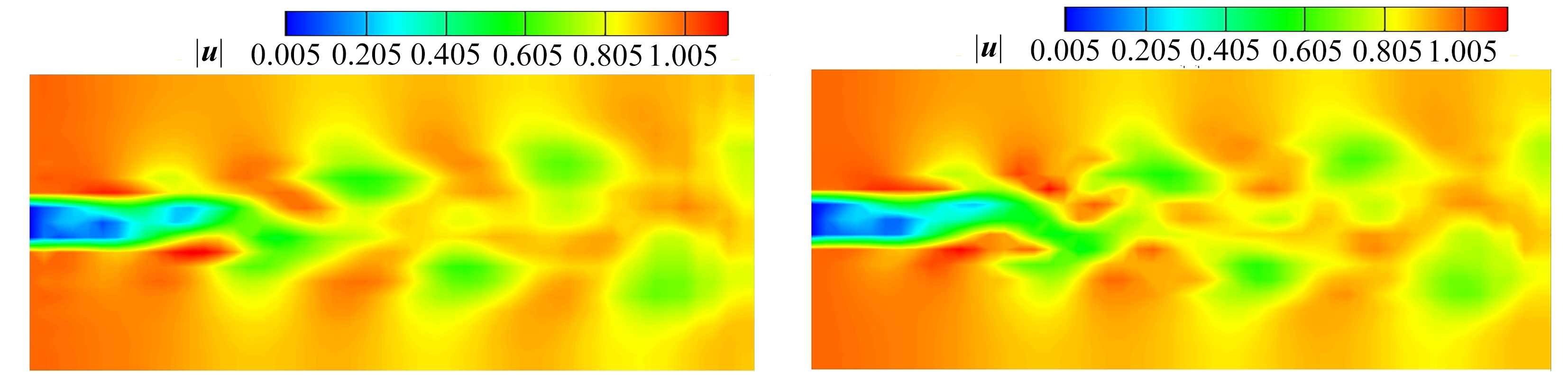(c) t=713.0最优动力系统流场 (d) t=713.0数据库流场 (c) The optimal dynamical system for t=713.0 (d) The database for t=713.0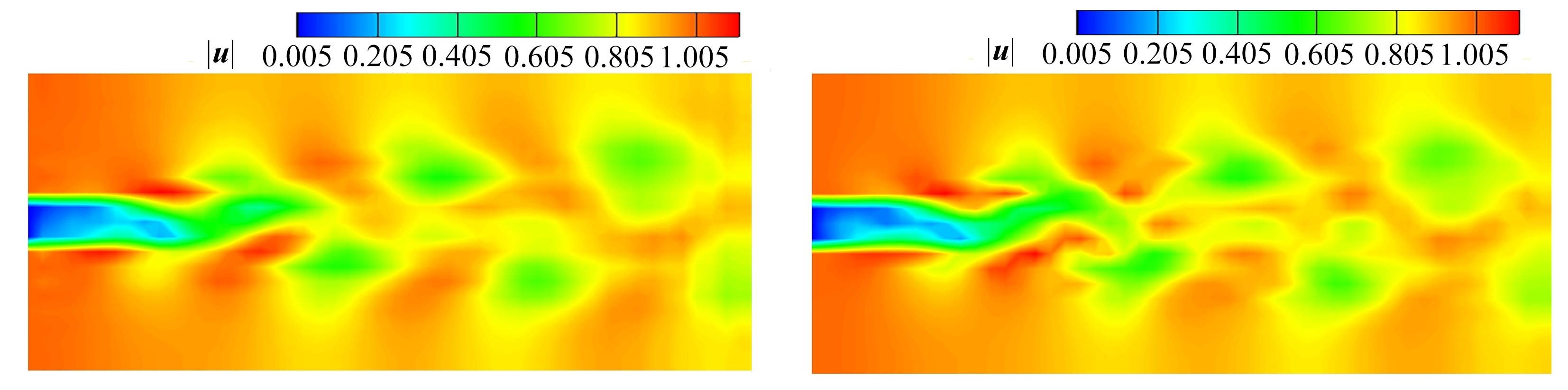(e) t=720.0最优动力系统流场 (f) t=720.0数据库流场 (e) The optimal dynamical system for t=720.0 (f) The database for t=720.0

Fig. 4 The comparison of the velocity magnitudes between the optimal dynamical system and the database

## 4 最优动力系统的动力学特性分析

### 4.1 相空间轨道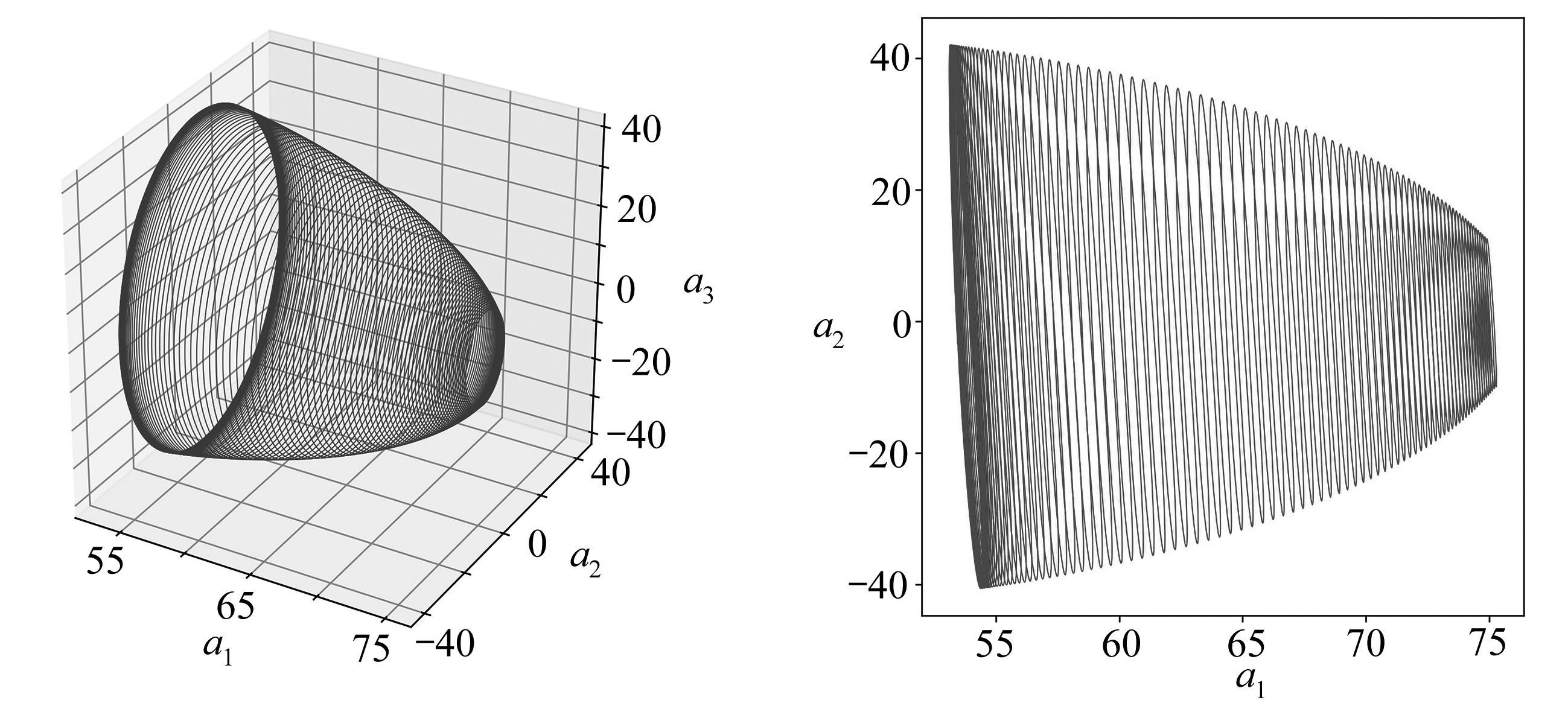(a) a1-a2-a3 (b) a1-a2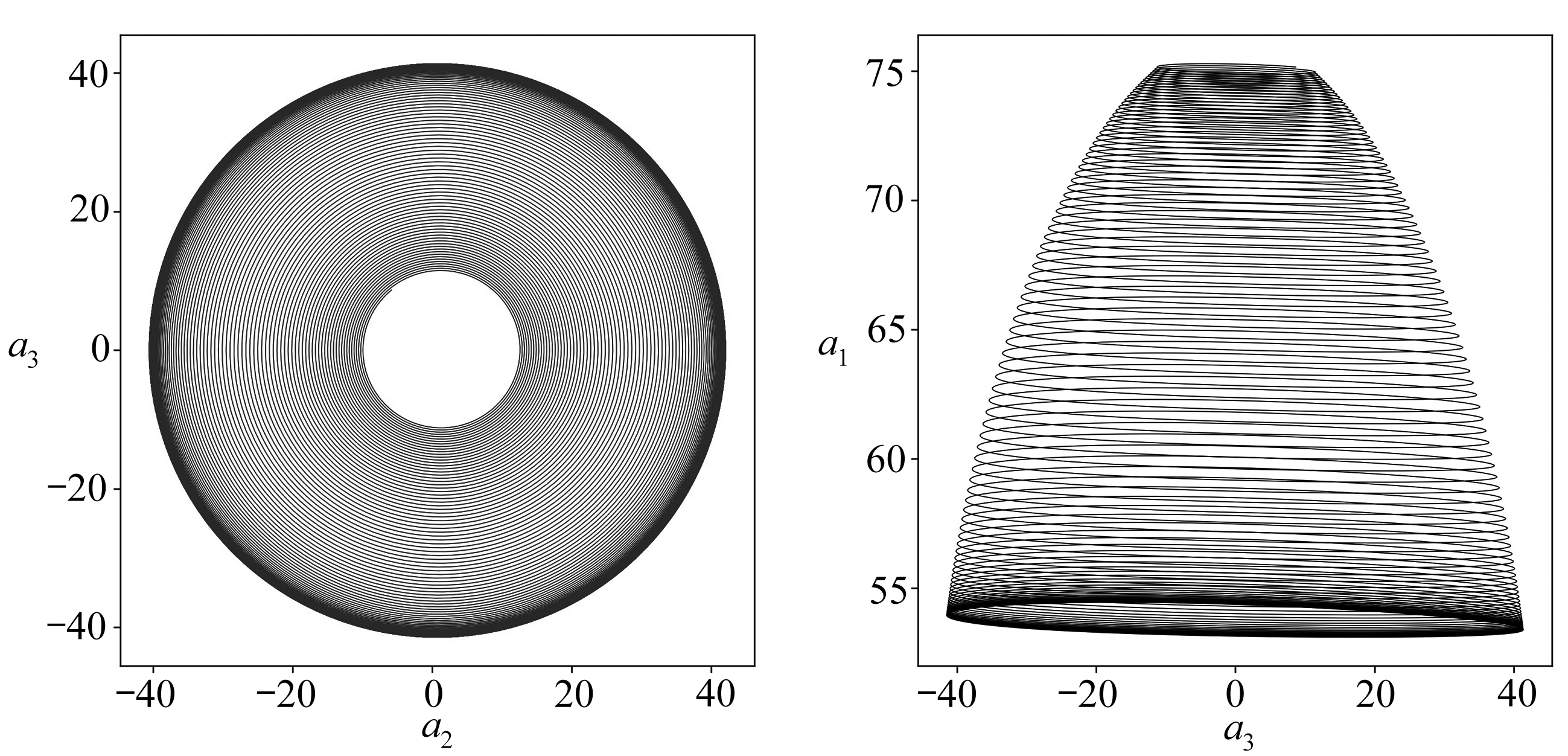(c) a2-a3 (d) a3-a1

Fig. 5 Phase portaits of the optimal dynamical system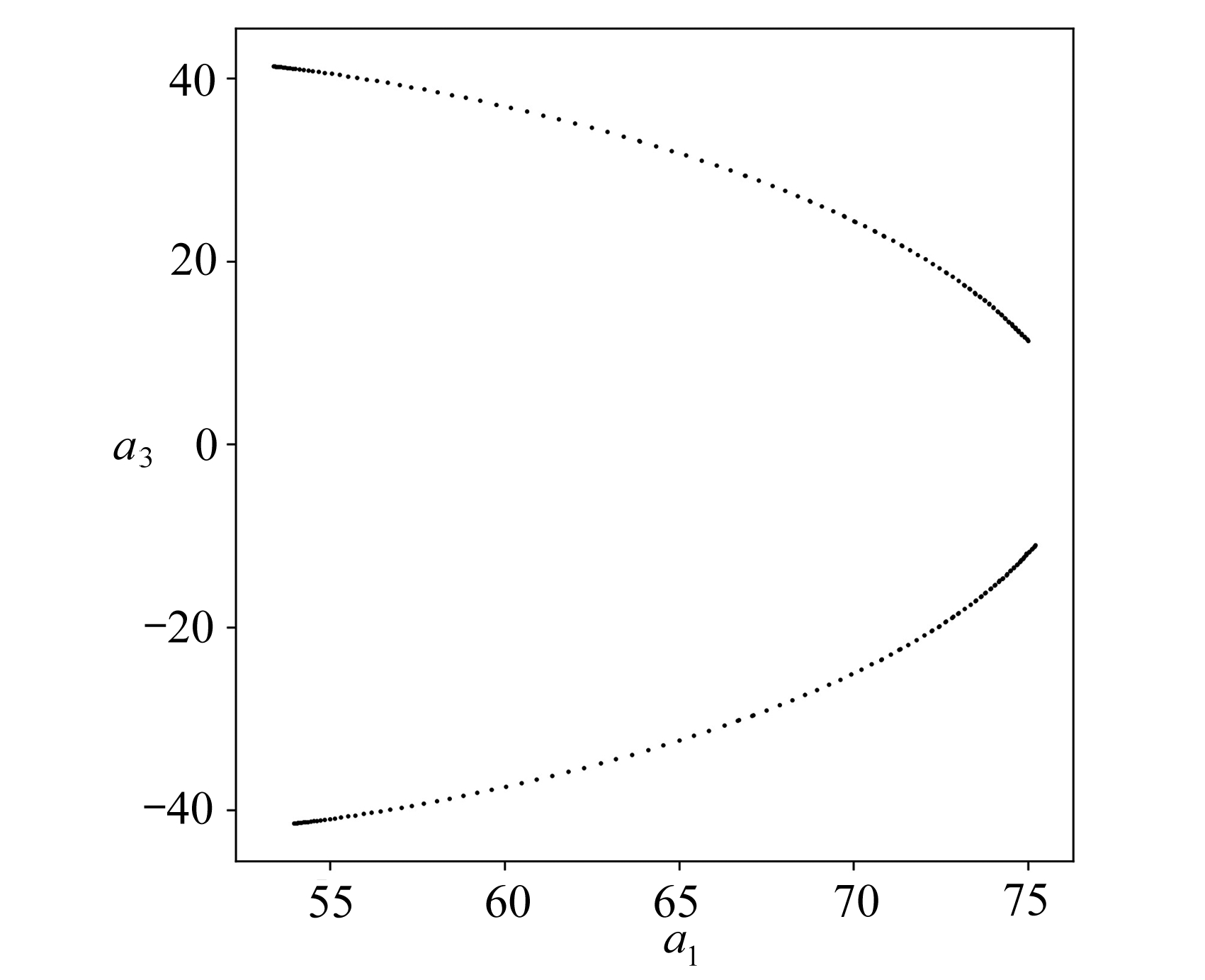Fig. 6 The Poincaré section formed with the phase portrait intersecting plane a2=0

### 4.2 Poincaré截面

Poincaré截面是一种分析高维动力系统的有效方法，可以看作是一种离散的动力系统.图6是最优动力系统在相平面a2=0上的Poincaré截面，右端的点对应初始时刻，左端的点对应于末端时刻，结合图5的相轨迹，可以断定此系统在Re=100时的长期动力学特性是极限环.

### 4.3 功率谱分析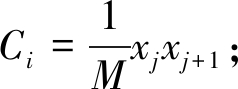(34)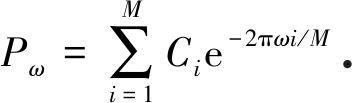(35)

### 4.4 Lyapunov指数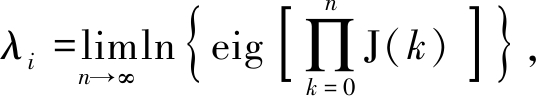(36)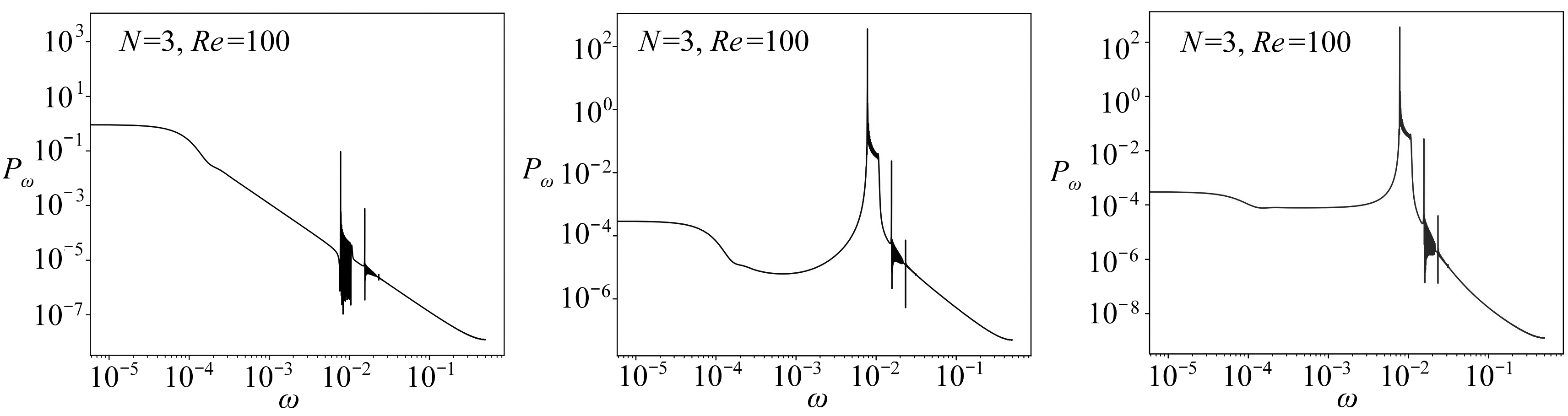(a) a1的功率谱 (b) a2的功率谱 (c) a3的功率谱 (a) The power spectrum of a1(b) The power spectrum of a2(c) The power spectrum of a3

Fig. 7 The power spectra of the portraits of optimal dynamical systems

Lyapunov指数的计算可以根据动力系统方程的右端项计算出Jacobi行列式而直接求出，但对于本文的最优动力系统，Jacobi行列式无法直接求出，这是因为对于为了使动力系统在任意时刻都满足边界条件，其右端项是随时间变化的，且不易求导，因此采用中心差分公式来计算Jacobi行列式，即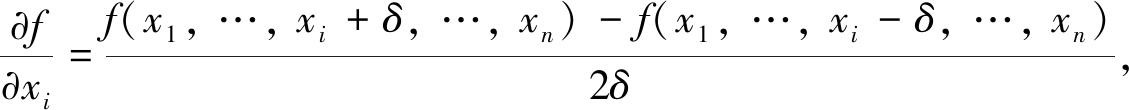(37)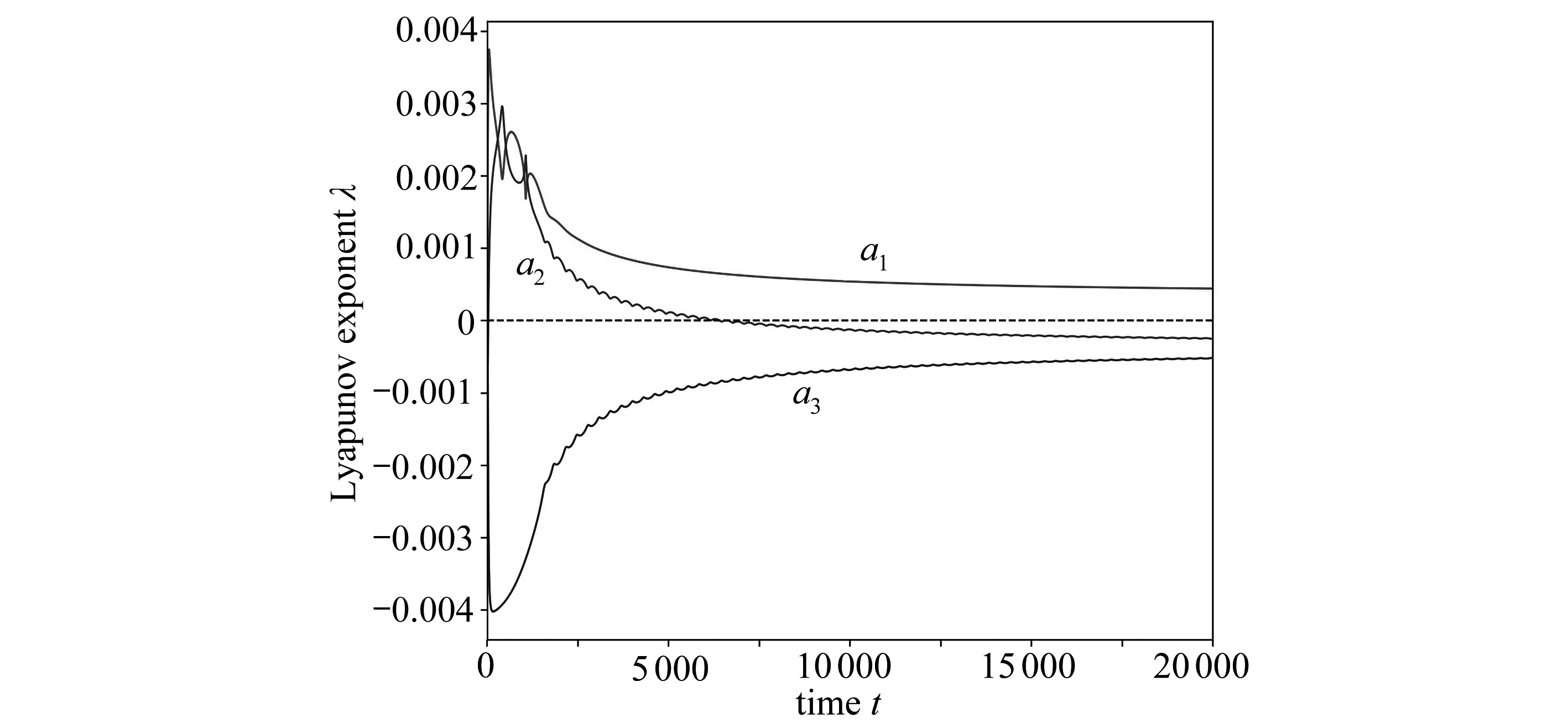Fig. 8 The evolution of the Lyapunov exponent spectrum of the optimal dynamical system

### 4.5 分岔特性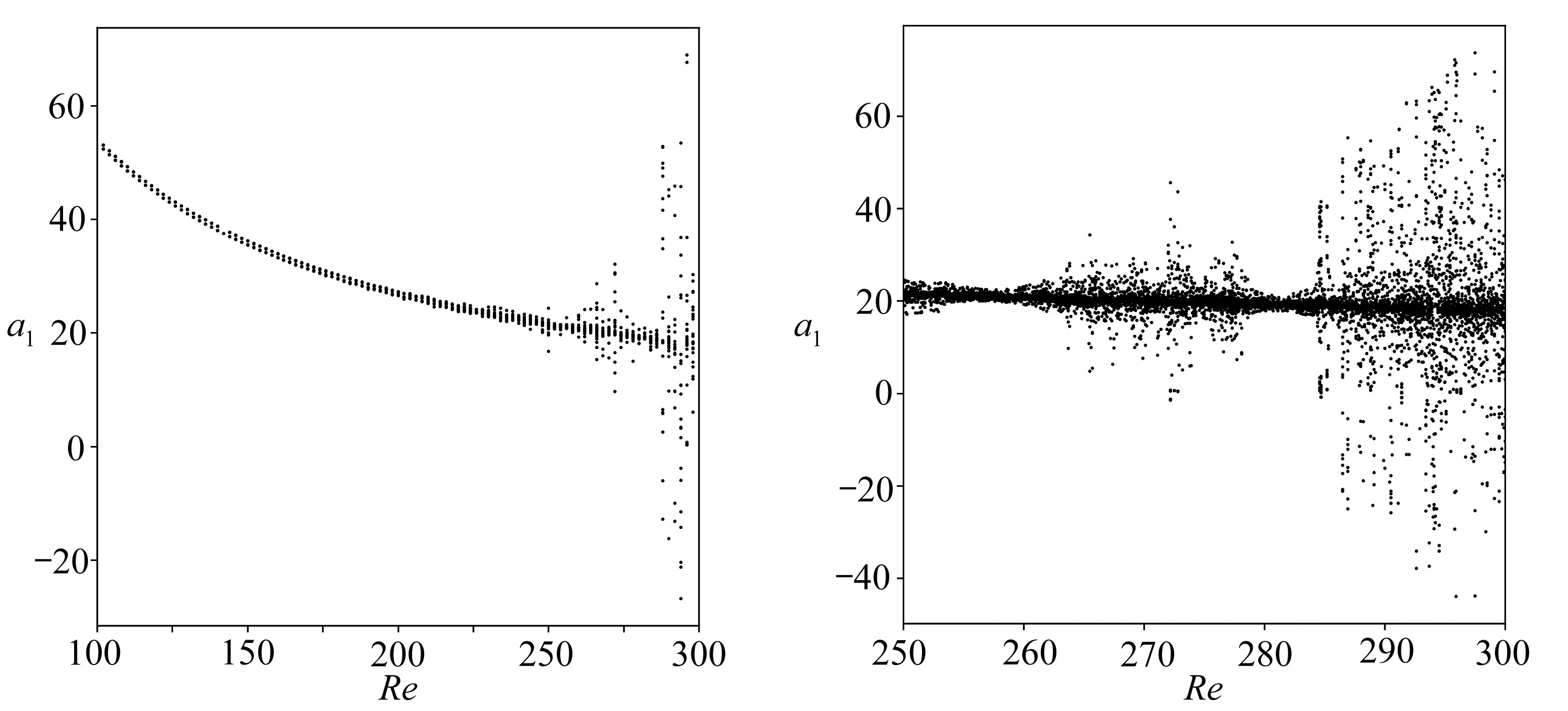(a) Re=100～300 (b) Re=250～300

Fig. 9 The bifurcation diagrams of the optimal system

## 5 结 论

 POPE S B. Turbulent Flows[M]. Cambridge: Cambridge University Press, 2000.

 HOLMES P, LUMLEY J L, BERKOOZ G. Turbulence, Coherent Structures, Dynamical Systems and Symmetry[M]. Cambridge: Cambridge University Press, 1996.

 是勋刚. 湍流[M]. 天津: 天津大学出版社, 1994.(SHI Xungang. Turbulence[M] Tianjin: Tianjin University Press, 1994.(in Chinese))

 SHRIER H N. Nonlinear Hydrodynamic Modeling: a Mathematical Introduction[M]. Berlin: Springer-Verlag, 1987.

 DANIELSON T J, OTTINO J M. Structural stability in two-dimensional model flows: Lagrangian and Eulerian turbulence[J]. Physics of Fluids A: Fluid Dynamics, 1990, 2(11): 2024-2035.

 POJE C, LUMLEY J L. A model for large scale structures in turbulence shear flows[J]. Journal of Fluid Mechanics, 1995, 285: 349-369.

 BERKOOZ G, HOLMES P, LUMLEY J L. The proper orthogonal decomposition in analysis of turbulent flows[J]. Annual Review of Fluid Mechanics, 1993, 25(1): 539-575.

 KALASHNIKOVA I, WAANDERS B V, ARUNAJATESAN S, et al. Stabilization of projection-based reduced order models for linear time-invariant systems via optimization-based eigenvalue reassignment[J]. Computer Methods in Applied Mechanics and Engineering, 2014, 272: 251-270.

 LUO Z D, YANG X Z, ZHOU Y J. A reduced finite difference scheme based on singular value decomposition and proper orthogonal decomposition for Burgers equation[J]. Journal of Computational and Applied Mathematics, 2009, 229(1): 97-107.

 ALONSO D, VELAZQUEZ A, VEGA J M. A method to generate computationally efficient reduced order models[J]. Computer Methods in Applied Mechanics and Engineering, 2009, 198(33/36): 2683-2691.

 WANG X L, JIANG Y L. Model order reduction methods for coupled systems in the time domain using Laguerre polynomials[J]. Computers and Mathematics With Applications, 2011, 62(8): 3241-3250.

 AMMAR A, MOKDAD B, CHINESTA F, et al. A new family of solvers for some classes of multidimensional partial differential equations encountered in kinetic theory modeling of complex fluids[J]. Journal of Non-Newtonian Fluid Mechanics, 2006, 139(3): 153-176.

 WU C J. Large optimal truncated low-dimensional dynamical systems[J]. Discrete and Continuous Dynamical Systems (Series A), 1996, 2(4): 559-583.

 WU C J, SHI H S. An optimal theory for an expansion of flow quantities to capture the flow structures[J]. Fluid Dynamics Research, 1995, 17(2): 67-85.

 吴锤结, 赵红亮. 不依赖数据库的最优动力系统建模理论及其应用[J]. 力学学报, 2001, 33(3): 289-300.(WU Chuijie, ZHAO Hongliang. A new database-free method of constructing optimal low-dimensional dynamical systems and its application[J] Acta Mechanica Sinica, 2001, 33(3): 289-300.(in Chinese))

 WU C J, WANG L. A method of constructing a database-free optimal dynamical system and a global optimal dynamical system[J]. Science in China(Series G): Physics, Mechanics & Astronomy, 2008, 51(7): 905-915.

 PENG N F, GUAN H, WU C J. Research on the optimal dynamical systems of three-dimensional Navier-Stokes equations based on weighted residual[J]. Science China: Physics, Mechanics & Astronomy, 2016, 59(4): 644-701.

 PENG N F, GUAN H, WU C J. Optimal dynamical systems of Navier-Stokes equations based on generalized helical-wave bases and the fundamental elements of turbulence[J]. Science China: Physics, Mechanics & Astronomy, 2016, 59(11): 114713. DOI: 10.1007/s11433-016-0247-3.

 TÖRN A, ZILINSKAS A. Global Optimization[M]. Berlin: Springer-Verlag, 1989.

 叶庆凯, 王肇明. 优化与最优控制中的计算方法[M]. 北京: 科学出版社, 1986.(YE Qingkai, WANG Zhaoming. Computational Methods of Optimization and Optimum Control[M]. Beijing: Science Press, 1986.(in Chinese))

 黄克智, 薛明德, 陆明万. 张量分析[M]. 北京: 清华大学出版社, 2003.(HUANG Kezhi, XUE Mingde, LU Mingwan. Tensor Analysis[M]. Beijing: Tsinghua University Press, 2003.(in Chinese))

 TORREY M D, MJOLSNESS R C, STEIN L R. NASA-VOF3D: a three-dimensional computer program for incompressible flows with free surfaces[R]. Nasa Sti/Recon Technical Report N, 1987.

 SIROVICH L. Turbulence and the dynamics of coherent structures, part Ⅲ: dynamics and scaling[J]. Quarterly of Applied Mathematics, 1987, 45(3): 561-571.

 CARBONE F, AUBRY N. Hierarchical order in wall-bounded shear turbulence[J]. Physics of Fluids, 1996, 8(4): 1061-1075.

 KAUFMAN E H, LEEMING D J, TAYLOR G D. An ODE-based approach to nonlinearly constrained minimax problems[J]. Numerical Algorithms, 1995, 9(1): 25-37.

# Analysis and Modelling of Optimal Dynamical Systems of Incompressible Navier-Stokes Equations

WANG Jincheng1, QI Jin2, WU Chuijie1

(1. School of Aeronautics and Astronautics, Dalian University of Technology,Dalian, Liaoning 116024, P.R.China;2. Institute of Applied Physics and Computational Mathematics, Beijing 100088, P.R.China)

Abstract: The modeling method for the optimal dynamical systems of Navier-Stokes equations satisfying both arbitrary velocity boundary conditions and velocity incompressible conditions was studied. Through the modeling and analysis of the optimal dynamical systems of the flow around the square column, it is found that the dynamics characteristics of the optimal dynamical systems are limit cycles. At the same time, the optimal dynamical system with only 3 optimal basis functions could well describe all the main flow field characteristics and the dynamics characteristics of the problem, so the proposed method is applicable to complex flow problems and their dynamics with minimal basis functions.

Key words: optimization; dynamical system; 3D Navier-Stokes equation; dynamics characteristics

ⓒ 应用数学和力学编委会，ISSN 1000-0887

http://www.applmathmech.cn

*收稿日期： 2019-09-06；

(我刊编委吴锤结来稿)

DOI: 10.21656/1000-0887.400279

(Contributed by WU Chuijie, M. AMM Editorial Board)

Foundation item: The National Natural Science Foundation of China(11601033; 11372068); The National Basic Research Program of China(973 Program)(2014CB744104)